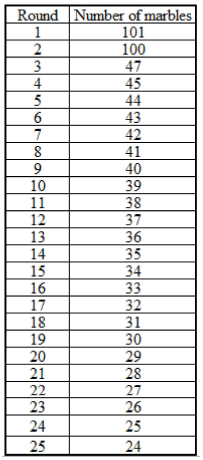Abhishek was playing an interesting timed game in which he was supposed to pick up the marbles scattered on the floor in exactly 5 minutes from the time the buzzer went off. During each of his 25 attempts, he tried to pick up the maximum number of marbles lying on the floor. However, after a certain number of attempts, he forgot the count of marbles he had picked up in each of the previous rounds. The only figure he could remember now was that he had picked up a total of 337 marbles in the first 5 rounds. Further, he never picked up the same number of marbles in any of the rounds and always picked a number of marbles less than the number of marbles picked up by him in the previous round.

Q. If in two of the rounds he had picked up 100 or more marbles, the least number of marbles he could have picked up in any of the rounds can be at most:

### Related TestManjit Kaur Jun 30, 2020Since We are supposed to maximize the least number of marbles he could have picked up, let us begin by assuming first that the least possible number of marbles he could have picked up in first two rounds was 201, (1st round: 101 and 2nd round: 100).
Now, in the next three rounds he would have picked up 136 marbles (337 - 101). To maximize the least number of marbles, the number of marbles he might have picked up in 3rd, 4th and 5th round should be as close as possible.
This gives us possible breakup of 47, 45 and 44 marbles respectively.
Now, from round 6 the number of marbles that he could have picked up can be in decreasing order (decremented by 1 at each step) as shown.The least number of marbles that he could have picked up in any of the rounds can be at most 24.